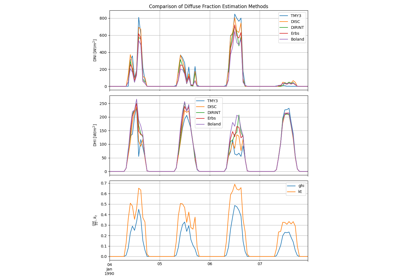Estimate DNI and DHI from GHI using the Erbs model.

The Erbs model 1 estimates the diffuse fraction DF from global horizontal irradiance through an empirical relationship between DF and the ratio of GHI to extraterrestrial irradiance, Kt. The function uses the diffuse fraction to compute DHI as

$DHI = DF \times GHI$

DNI is then estimated as

$DNI = (GHI - DHI)/\cos(Z)$

where Z is the zenith angle.

Parameters
• ghi (numeric) – Global horizontal irradiance in W/m^2.

• zenith (numeric) – True (not refraction-corrected) zenith angles in decimal degrees.

• datetime_or_doy (int, float, array, pd.DatetimeIndex) – Day of year or array of days of year e.g. pd.DatetimeIndex.dayofyear, or pd.DatetimeIndex.

• min_cos_zenith (numeric, default 0.065) – Minimum value of cos(zenith) to allow when calculating global clearness index kt. Equivalent to zenith = 86.273 degrees.

• max_zenith (numeric, default 87) – Maximum value of zenith to allow in DNI calculation. DNI will be set to 0 for times with zenith values greater than max_zenith.

Returns

data (OrderedDict or DataFrame) –

Contains the following keys/columns:

• dni: the modeled direct normal irradiance in W/m^2.

• dhi: the modeled diffuse horizontal irradiance in W/m^2.

• kt: Ratio of global to extraterrestrial irradiance on a horizontal plane.

References

1

D. G. Erbs, S. A. Klein and J. A. Duffie, Estimation of the diffuse radiation fraction for hourly, daily and monthly-average global radiation, Solar Energy 28(4), pp 293-302, 1982. Eq. 1

## Examples using pvlib.irradiance.erbs#Diffuse Fraction Estimation

Diffuse Fraction Estimation